Next: Worked example 5.5: Sliding Up: Conservation of energy Previous: Worked example 5.3: Stretching

## Worked example 5.4: Roller coaster ride

Question: A roller coaster cart of mass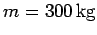starts at rest at point, whose height off the ground is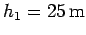, and a little while later reaches point, whose height off the ground is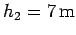. What is the potential energy of the cart relative to the ground at point? What is the speed of the cart at point, neglecting the effect of friction?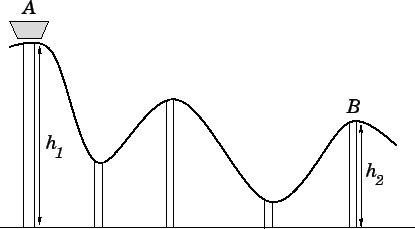Answer: The gravitational potential energy of the cart with respect to the ground at pointis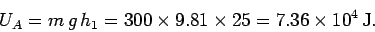Likewise, the potential energy of the cart at pointis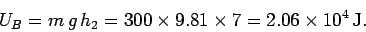Hence, the change in the cart's potential energy in moving from pointto pointis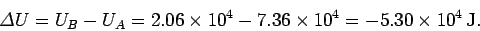By energy conservation,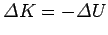, whererepresents kinetic energy. However, since the initial kinetic energy is zero, the change in kinetic energy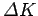is equivalent to the final kinetic energy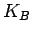. Thus,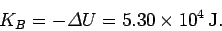Now,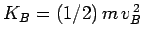, where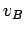is the final speed. Hence,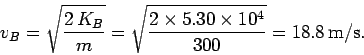Next: Worked example 5.5: Sliding Up: Conservation of energy Previous: Worked example 5.3: Stretching
Richard Fitzpatrick 2006-02-02